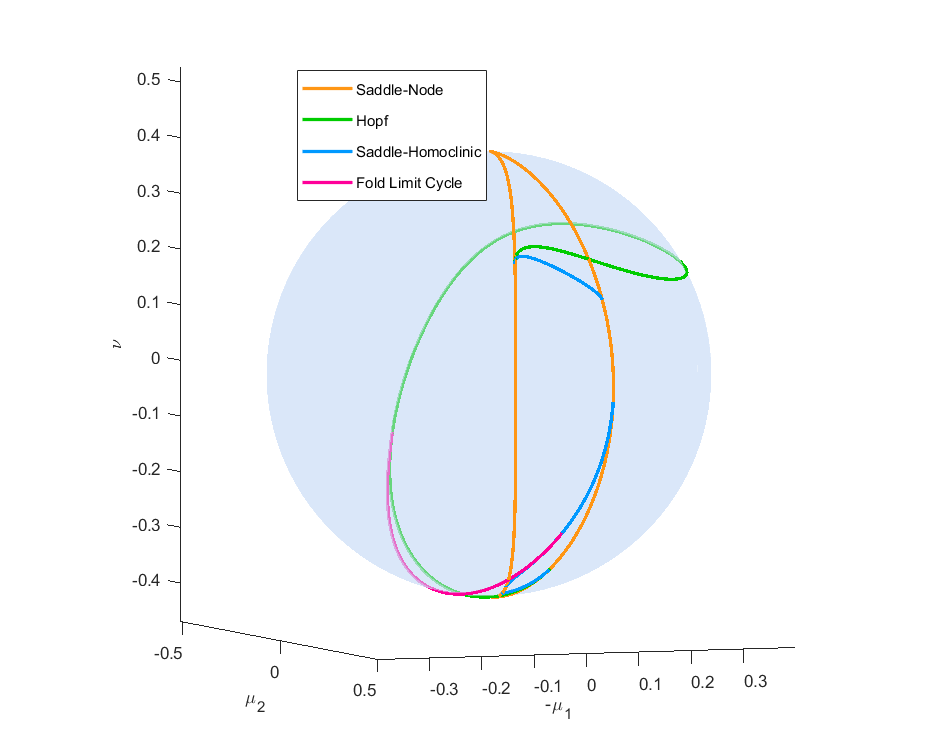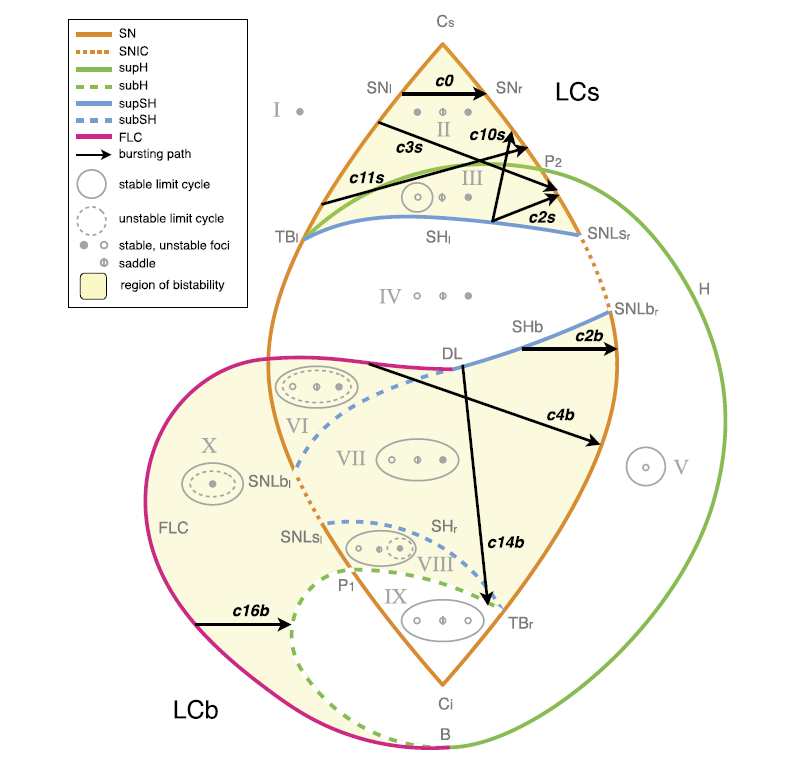In :
%matplotlib widget
import matplotlib.pyplot as plt
import numpy as np
from tvb.simulator.lab import *
from tvb.simulator.plot.phase_plane_interactive import PhasePlaneInteractive
from tvb.simulator.models.epileptorcodim3 import EpileptorCodim3
from tvb.simulator.models.epileptorcodim3 import EpileptorCodim3SlowMod
from mpl_toolkits.mplot3d import Axes3D

from tvb.simulator.plot.epileptor_plotter import EpileptorModelPlot

WARNING  File 'hemispheres' not found in ZIP.


# Epileptor codim 3 model¶

The Epileptor codim 3 model, developed by Saggio et al. 2017, is a neural mass model which has a rich set of possible bursting patterns. These bursters correspond to a seizure-like state as recorded in SEEG-recordings of epileptic patients. In this tutorial we will explore a number of classes of bursting which are present in this model.

The model consists of a fast and a slow subsystem. The fast subsystem is based on a spherical unfolding of the degenerate Takens-Bogdanov bifurcation. The slow subsystem controls the parameters of the fast subsystem along a path on the spherical unfolding. The class of a burster is determined by which bifurcations happen at onset and offset of the oscillation, which is in turn determined by the path on the spherical unfolding.

In :
Epileptorcd3 = EpileptorCodim3()
ep = EpileptorModelPlot()


First we look at the phase plane. The default parameters of the model are setup so we have a Saddle-Node bifurcation at onset and a Saddle-Homoclinic bifucation at offset. This corresponds to bifurcations in the original Epileptor model. By adjusting the variable z, we can travel on the spherical unfolding in a predetermined path. The Saddle-Node bifurcation happens at z=0 and the Saddle Homoclinic bifurcation happens at z=0.14.

In :
Epileptorcd3.state_variable_range["x"] = np.array([-2.0, 2.0])
Epileptorcd3.state_variable_range["y"] = np.array([-2.0, 2.0])
Epileptorcd3.state_variable_range["z"] = np.array([-0.1, 0.3])
ppi_fig = PhasePlaneInteractive(model=Epileptorcd3)
ppi_fig.show()
Epileptorcd3.state_variable_range["x"] = np.array([0.4, 0.6])
Epileptorcd3.state_variable_range["y"] = np.array([-0.1, 0.1])
Epileptorcd3.state_variable_range["z"] = np.array([0.0, 0.1])


Next we simulate the model to validate that the desired burster happens.

In :
ep.show()

WARNING  random_state supplied for non-stochastic integration


# Classes of bursters¶

As stated earlier, the class of burster is affected by which path the bifurcation parameters take on the spherical unfolding of the degenerate Takens-Bogdanov bifurcation. These figures, from Saggio et al. 2017, show the bifurcation diagram of the deg. TB-bifurcation of the focus type. We parametrise theses paths using an arc of a great circle.Here are the parameter settings for the bursting classes which are listed above. They are constructed by choosing the vectors A and B as the starting and end point of the arc of the great circle respectively. Note that the bursters happen at different timescales.

This is a simulation of the 'c0' class which is a Saddle-Node/Saddle-Node burster. While this class behaves like a buster, it does not contain a limit cycle during the oscillatory phase.

In :
A = [0.2649, -0.05246, 0.2951]
B = [0.2688, 0.05363, 0.2914]
c = 0.001
ep.show(burster_class='c0')

WARNING  random_state supplied for non-stochastic integration
WARNING  random_state supplied for non-stochastic integration


This is a simulation of the 'c2s' class which is a Saddle-Node/Saddle-Homoclinic burster. This is the default setting for the Epileptor codim 3 model and is the same burster which is the basis of the original Epileptor model.

In :
A = [0.3448,0.02285,0.2014]
B = [0.3351,0.07465,0.2053]
c = 0.001

ep.show(burster_class='c2s')

WARNING  random_state supplied for non-stochastic integration


This is a simulation of the 'c3s' class which is a Saddle-Node/Supercritical Hopf burster.

In :
A = [0.2552,-0.0637,0.3014]
B = [0.3496,0.0795,0.1774]
c = 0.0004

ep.show(burster_class='c3s')

WARNING  random_state supplied for non-stochastic integration
WARNING  random_state supplied for non-stochastic integration


This is a simulation of the 'c10s' class which is a Supercritical Hopf/Saddle-Homoclinic burster.

In :
A = [0.3448,0.0228,0.2014]
B = [0.3118,0.0670,0.2415]
c = 0.00005

ep.show(burster_class='c10s')

WARNING  random_state supplied for non-stochastic integration
WARNING  random_state supplied for non-stochastic integration


This is a simulation of the 'c11s' class which is a Saddle-Node/Saddle-Node burster.

In :
A = [0.3131,-0.06743,0.2396]
B = [0.3163,0.06846,0.2351]
c = 0.00004

ep.show(burster_class='c11s')

WARNING  random_state supplied for non-stochastic integration
WARNING  random_state supplied for non-stochastic integration


This is a simulation of the 'c2b' class which is a Saddle-Node/Saddle-Homoclinic burster. It is similar to the 'c2s' class, but it has a big limit cycle which encompasses the stable node.

In :
A = [0.3216,0.0454,-0.2335]
B = [0.285,0.05855,-0.2745]
c = 0.004

ep.show(burster_class='c2b')

WARNING  random_state supplied for non-stochastic integration


This is a simulation of the 'c4b' class which is a Saddle-Node/Fold Limit Cycle burster with a big limit cycle.

In :
A = [0.1871,-0.02512,-0.3526]
B = [0.2081,-0.01412,-0.3413]
c = 0.008

ep.show(burster_class='c4b')

WARNING  random_state supplied for non-stochastic integration


This is a simulation of the 'c14b' class which is a Subcritical Hopf/Saddle-Homoclinic burster with a big limit cycle.

In :
A = [0.3216,0.0454,-0.2335]
B = [0.106,0.005238,-0.3857]
c = 0.002

ep.show(burster_class='c14b')

WARNING  random_state supplied for non-stochastic integration
WARNING  random_state supplied for non-stochastic integration


This is a simulation of the 'c16b' class which is a Subcritical/Fold Limit Cycle burster with a big limit cycle.

In :
A = [0.04098,-0.07373,-0.391]
B = [-0.01301,-0.03242,-0.3985]
c = 0.004

ep.show(burster_class='c16b')

WARNING  random_state supplied for non-stochastic integration


It is also possible to use spherical coördinates (R, phi, theta) to design a great circle. Here R is the radius of the sphere, phi is the azimuthal angle and theta is the polar angle. The corresponding Cartesian coordinates are (mu2,-mu1,nu)

In :
Epileptorcd3=EpileptorCodim3()

phi_start = -0.2
theta_start = 0.93
phi_stop = np.pi/4
theta_stop = 0.2
R = 0.4 #Default radius of the sphere

Epileptorcd3.mu2_start=np.array([R*np.sin(theta_start)*np.cos(phi_start)])
Epileptorcd3.mu1_start=np.array([-R*np.sin(theta_start)*np.sin(phi_start)])
Epileptorcd3.nu_start=np.array([R*np.cos(theta_start)])
Epileptorcd3.mu2_stop=np.array([R*np.sin(theta_stop)*np.cos(phi_stop)])
Epileptorcd3.mu1_stop=np.array([-R*np.sin(theta_stop)*np.sin(phi_stop)])
Epileptorcd3.nu_stop=np.array([R*np.cos(theta_stop)])
Epileptorcd3.R=np.array([R])
Epileptorcd3.c=np.array([0.001])

Epileptorcd3.variables_of_interest=['x', 'y', 'z']
sim = simulator.Simulator(
model=Epileptorcd3,
connectivity=connectivity.Connectivity.from_file(),
coupling=coupling.Linear(a=np.array([0.0152])),
integrator=integrators.HeunDeterministic(dt=2 ** -4),
monitors=(monitors.TemporalAverage(period=2 ** -2),),
simulation_length=2 ** 12,
).configure()
(tavg_time, tavg_data), = sim.run()
plt.figure()
plt.plot(tavg_time, tavg_data[:, 0, 0, 0], label='x')
plt.plot(tavg_time, tavg_data[:, 2, 0, 0], label='z')
plt.legend()
plt.grid(True)
plt.xlabel('Time (ms)')
plt.ylabel("Temporal average")

fig2 = plt.figure()
ax.plot(tavg_data[:, 0, 0, 0], tavg_data[:, 1, 0, 0], tavg_data[:, 2, 0, 0])
plt.grid(True)
plt.xlabel('x')
plt.ylabel('y')
plt.show()

WARNING  File 'hemispheres' not found in ZIP.
WARNING  random_state supplied for non-stochastic integration


# Epileptogenicity and modification¶

The value of c determines the size of the timescale separation between the slow and the fast subsystem. For timescale separation we need that c<< 1 , though it depends on the bifurcations what value of c is suitable. The epileptogenicity of the system is determined by the value of dstar. For dstar>1 (approximately) the system will get stuck in the bursting state.

The default value for dstar is 0.3 which produces the usual pattern of stability followed by bursters

In :
dstar = np.array([0.3])

ep.show()

WARNING  random_state supplied for non-stochastic integration


For dstar equal to 0.1 the oscillations are shorter and less frequent than the default value. Though for every positive value of dstar we still have bursting activity.

In :
dstar = np.array([0.1])

ep.show()

WARNING  random_state supplied for non-stochastic integration


If dstar is 0 then the system remains stable.

In :
dstar = np.array()

ep.show()

WARNING  random_state supplied for non-stochastic integration


For dstar larger than 1 (approximately) the system will head to a indefinite oscillatory state.

In :
dstar = np.array()

ep.show()

WARNING  random_state supplied for non-stochastic integration


For a negative dstar the bifurcation parameters wraps around the great circle in the wrong direction, producing an artificial burster

In :
dstar = np.array([-0.3])

ep.show()

WARNING  random_state supplied for non-stochastic integration


By introducing a small modification it is possible to stabilize the system without affecting the model with a positive dstar

In :
dstar = np.array()
modification = np.array([True])

ep.show()

WARNING  random_state supplied for non-stochastic integration


Here we see that the modification doesn't affect the generic oscillations of the system. Though note that the initial conditions are random.

In :
#Unmodified
ep.show()

WARNING  random_state supplied for non-stochastic integration

In :
#Modified
ep.show()

WARNING  random_state supplied for non-stochastic integration

c:\work\tvb\tvb-root\scientific_library\tvb\simulator\plot\epileptor_plotter.py:311: RuntimeWarning: More than 20 figures have been opened. Figures created through the pyplot interface (matplotlib.pyplot.figure) are retained until explicitly closed and may consume too much memory. (To control this warning, see the rcParam figure.max_open_warning).
self.fig = plt.figure(figsize=(9,5))


# Ultra-slow transition of classes of bursters¶

By adjusting the path through the parameter space we can transition between classes of bursters. This is implemented in the EpileptorCodim3_slowmod model. Most input parameters are the same as the EpileptorCodim3 model. However the input parameters which dictate the class of burster has been modified. The offset and onset parameters are given of the initial class of burster are given by the subscript Ain and Bin respectively. The offset and onset parameters are given of the final class of burster are given by the subscript Aend and Bend respectively.

In :
EpileptorCodim3SlowMod()

Out:

### EpileptorCodim3SlowMod

value
Ks [min, median, max]
[0, 0, 0]
Ks dtype
float64
Ks shape
(1,)
N [min, median, max]
[1, 1, 1]
N dtype
int32
N shape
(1,)
R [min, median, max]
[0.4, 0.4, 0.4]
R dtype
float64
R shape
(1,)
Type
EpileptorCodim3SlowMod
b [min, median, max]
[1, 1, 1]
b dtype
float64
b shape
(1,)
c [min, median, max]
[0.002, 0.002, 0.002]
c dtype
float64
c shape
(1,)
cA [min, median, max]
[0.0001, 0.0001, 0.0001]
cA dtype
float64
cA shape
(1,)
cB [min, median, max]
[0.00012, 0.00012, 0.00012]
cB dtype
float64
cB shape
(1,)
dstar [min, median, max]
[0.3, 0.3, 0.3]
dstar dtype
float64
dstar shape
(1,)
gid
UUID('45629417-343f-43b4-933c-d6f4a0ab8862')
modification dtype
bool
modification shape
(1,)
mu1_Aend [min, median, max]
[0.06485, 0.06485, 0.06485]
mu1_Aend dtype
float64
mu1_Aend shape
(1,)
mu1_Ain [min, median, max]
[0.05494, 0.05494, 0.05494]
mu1_Ain dtype
float64
mu1_Ain shape
(1,)
mu1_Bend [min, median, max]
[0.03676, 0.03676, 0.03676]
mu1_Bend dtype
float64
mu1_Bend shape
(1,)
mu1_Bin [min, median, max]
[-0.0461, -0.0461, -0.0461]
mu1_Bin dtype
float64
mu1_Bin shape
(1,)
mu2_Aend [min, median, max]
[0.07337, 0.07337, 0.07337]
mu2_Aend dtype
float64
mu2_Aend shape
(1,)
mu2_Ain [min, median, max]
[0.2731, 0.2731, 0.2731]
mu2_Ain dtype
float64
mu2_Ain shape
(1,)
mu2_Bend [min, median, max]
[-0.02792, -0.02792, -0.02792]
mu2_Bend dtype
float64
mu2_Bend shape
(1,)
mu2_Bin [min, median, max]
[0.243, 0.243, 0.243]
mu2_Bin dtype
float64
mu2_Bin shape
(1,)
nu_Aend [min, median, max]
[-0.3878, -0.3878, -0.3878]
nu_Aend dtype
float64
nu_Aend shape
(1,)
nu_Ain [min, median, max]
[0.287, 0.287, 0.287]
nu_Ain dtype
float64
nu_Ain shape
(1,)
nu_Bend [min, median, max]
[-0.3973, -0.3973, -0.3973]
nu_Bend dtype
float64
nu_Bend shape
(1,)
nu_Bin [min, median, max]
[0.3144, 0.3144, 0.3144]
nu_Bin dtype
float64
nu_Bin shape
(1,)
state_variable_range
{'x': array([0.4, 0.6]), 'y': array([-0.1,  0.1]), 'z': array([0. , 0.1]), 'uA': array([0., 0.]), 'uB': array([0., 0.])}
title
EpileptorCodim3SlowMod gid: 45629417-343f-43b4-933c-d6f4a0ab8862
variables_of_interest
('x', 'z')

The default setting corresponds to a transition from the classes c0, c11s, c10s, c2s, c2b, c4b and finally c16b. You might need to zoom in to clearly see the different bursters.

In :
ep.show()

WARNING  random_state supplied for non-stochastic integration